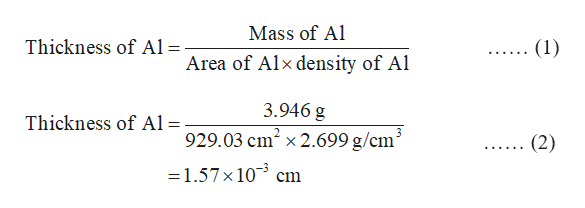# A sheet of aluminum (Al) foil has a total area of 1.000 ft2 and a mass of 3.946 g. What is the thickness of the foil in millimeters (density of Al = 2.699 g/cm3)?provide answer in mm

Question
290 views

A sheet of aluminum (Al) foil has a total area of 1.000 ft2 and a mass of 3.946 g.

What is the thickness of the foil in millimeters (density of Al = 2.699 g/cm3)?

check_circle

Step 1

The conversion factor for ft2 and cm2 units is:

1.0 ft2 = 929.03 cm2

Hence, the area of aluminium foil in cm2 units is 929.03 cm2.

Step 2

The formula for calculating thickness of aluminium foil is given in the equation (1).

The thickness of given aluminium foil is calculated in cm units in the equation (2).help_outlineImage TranscriptioncloseMass of Al Thickness of Al =- (1) Area of Alx density of Al 3.946 g Thickness of Al = 929.03 cm2 x 2.699 g/cm (2) =1.57x 10 cm fullscreen
Step 3

The conversion factor for centimeter and millimetre units is:

1 cm = 10 mm

Hence, 1.57 × 10-3 cm = 1.57 × 10-3 × 10 mm

...

### Want to see the full answer?

See Solution

#### Want to see this answer and more?

Solutions are written by subject experts who are available 24/7. Questions are typically answered within 1 hour.*

See Solution
*Response times may vary by subject and question.
Tagged in